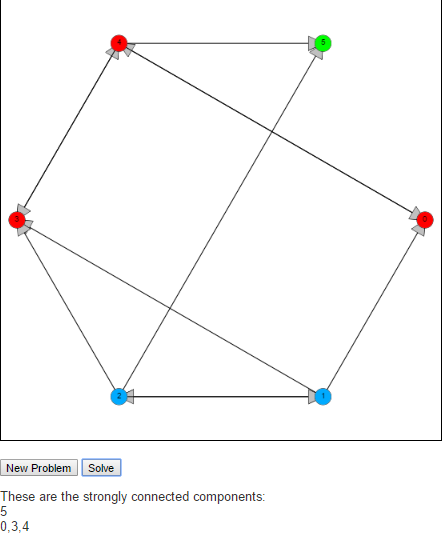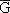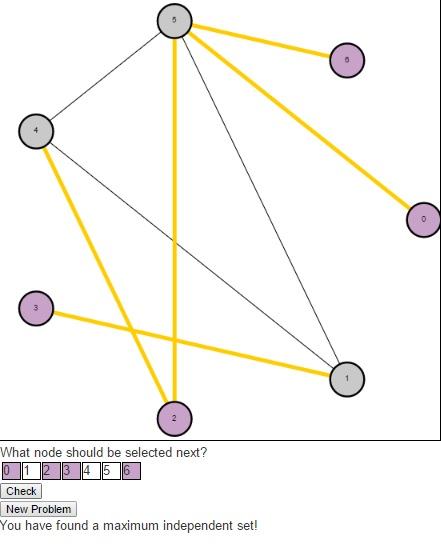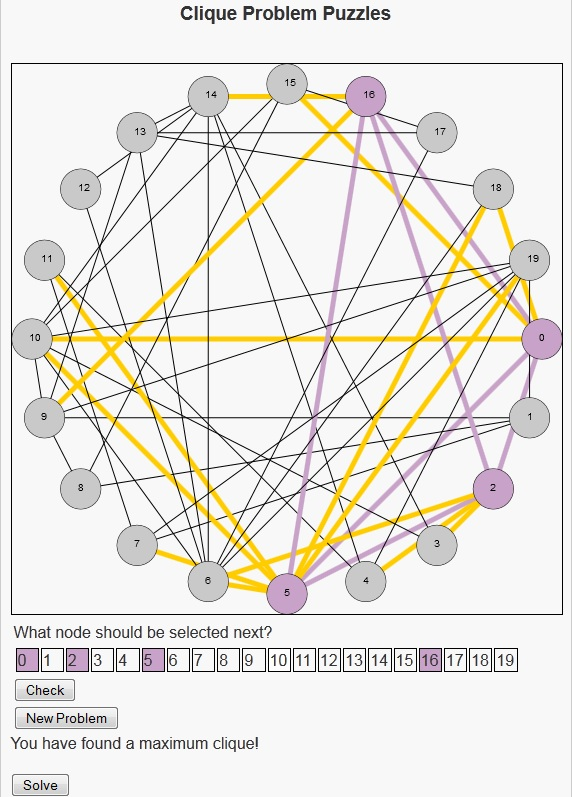# Tarjan’s Strongly Connected Components AlgorithmA strongly connected component of a graph is a subgraph S of G where every pair of nodes, u and v in S there is a path from u to v and a path from v to u.

To find these strongly connected components we implement Tarjan’s algorithm. The idea behind Tarjan’s algorithm is to begin by running a depth first search from an arbitrary node in the graph, labeling nodes reachable from this start node in the order they are reached. The algorithm is also interested in the “oldest” node that could be reached by a given node. This is indicated by the keeping track of the lowest label that can be reached from that node. We will call the first property label(v) and the second lowlink(v).

When the algorithm starts label(v) is the same as lowlink(v) whenever a node is discovered. As the algorithm is executed, the DFS is being run on each discovered node, which in turn updates the lowlink(v) property telling of (older) nodes that can be reached. If an older node can be reached, then we update lowlink. If we reach a node that cannot connect to any older nodes after the DFS call, i.e if label(v) is the same a lowlink(v), then this means that this node does not have a path to any node with a lower label. So this node will be the first node of a new strongly connected component.

Feel free to check it out an let me know what you think in the comments below.

# Independent Set Puzzles

In this post, I want to return to the idea of NP-Complete problems. There is a more technical, more formal definition that I can refer you to, but I like to refer to the images from Garey and Johnson’s “Computers and Intractability: A Guide to the Theory of NP Completeness”. The images helps to understand the difficulty of NP-Complete problems by presenting two images. The first image shows a single individual speaking to someone saying that he has been unable to solve the problem. The second image shows that same individual speaking to the same person behind the desk, but saying that not only was he unable to solve the problem but neither was a long line of people. The theory of NP Complete problems revolves around the concept that if an efficient algorithm exists for an NP-Complete problem, then an efficient algorithm exists for all problems in the class NP.

Today, I would like to present a puzzle I created to play with the Independent Set problem. This is the problem where we are given a graph G = (V, E) and are asked to find a maximum set of vertices S such that there is no edge in the graph G between any two vertices in S. The decision version (the problem asking whether there is an independent set of size k) of this problem is NP-Complete, so the known algorithms for problem either have a slow running time, or do not solve it exactly.

This problem is very related to another puzzle I posted last year called the clique problem. In fact, Karp originally proved that Clique was NP-Complete by showing that if Clique could be solved efficiently, then Independent Set could be solved efficiently. He did this by constructing a second graph [G bar], called the compliment of G (containing the same vertices in G, along with the edges that are not present in G. Any edge present in G will not be present in). Then he showed that the nodes representing a maximum clique in G would represent a maximum independent set in. He had already shown that Independent Set was NP-Complete, which meant that both Independent Set and Clique were among the most difficult problems within the class known as NP.The puzzle begins with an undirected graph and asks users to find a maximum independent set. Users should click on the numbers in the table below the graph indicating the nodes they wish to select in their independent set (purple indicates that the node is selected, gray indicates that it is not). Once a user have a potential solution, they can press the “Check” button to see if their solution is optimal. If a user is having trouble and simply wishes to see the maximum independent set, they can press the “Solve” button. And to generate a new problem, users can press the “New Problem” button.As a result of this relationship between the Clique problem and the Independent Set problem, the Bron-Kerbosch algorithm that was used to find maximum cliques previously can also be used here.

# Clique Problem Puzzles

I still remember how I felt when I was first introduced to NP-Complete problems. Unlike the material I had learned up to that point, there seemed to be such mystery and intrigue and opportunity surrounding these problems. To use the example from Garey and Johnson’s book “Computers and Intractability: A Guide to the Theory of NP Completeness”, these were problems that not just one researcher found difficult, but that a number of researchers had been unable to find efficient algorithms to solve them. So what they did was show that the problems all had a special relationship with one another, and thus through this relationship if someone were to discover an algorithm to efficiently solve any one of these problems they would be able to efficiently solve all the problems in this class. This immediately got my mind working into a world where I, as a college student, would discover such an algorithm and be mentioned with the heavyweights of computer science like Lovelace, Babbage, Church, Turing, Cook, Karp and Dean.

Unfortunately I was a student so I did not have as much time to devote to this task as I would have liked. In my spare time though I would try to look at problems and see what kind of structure I found. One of my favorite problems was, The Clique Problem. This is a problem where we are given an undirected graph and seek to find a maximum subset of nodes in this graph that all have edges between them, i.e. a clique (Actually the NP-Complete version of this problem takes as input an undirected graph G and an integer k and asks if there is a clique in G of size k).

Although I now am more of the mindset that there do not exist efficient algorithms to solve NP-Complete problems, I thought it would be a nice project to see if I could re-create this feeling – both in myself and others. So I decided to write a program that generates a random undirected graph and asks users to try to find a maximum clique. To test users answers, I coded up an algorithm that works pretty well on smaller graphs, the Bron-Kerbosch Algorithm. This algorithm uses backtracking to find all maximal cliques, which then allows us to sort them by size and determine the largest.Users should click on the numbers in the table below the canvas indicating the nodes they wish to select in their clique (purple indicates that the node is selected, gray indicates that it is not). Once they have a potential solution, they can press the “Check” button to see if their solution is optimal. If a user is having trouble and simply wishes to see the maximum clique, they can press the “Solve” button. And to generate a new problem, users can press the “New Problem” button.

So I hope users have fun with the clique problem puzzles, and who knows maybe someone will discover an algorithm that efficiently solves this problem and become world famous.

# Interactive Tutorial of Kruskal’s AlgorithmI just finished working on another implementation of Kruskal’s algorithm. This version depends more on user input to generate the minimum spanning path tree.

Check it out.

# Interactive Tutorial of Prim’s AlgorithmI just finished working on another implementation of Prim’s algorithm. This version depends more on user input to generate the minimum spanning path tree.

Check it out.